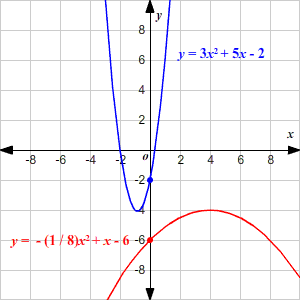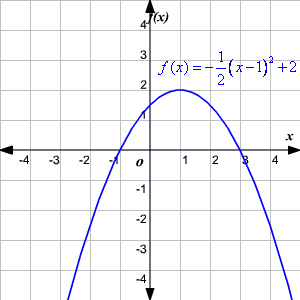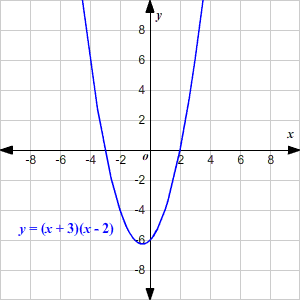A quadratic equation is a polynomial equation of degree $2$ .  The standard form of a quadratic equation is

$0=a{x}^{2}+bx+c$

where   $a,b$ and $c$ are all real numbers and $a\ne 0$ .

In this equation, $\left(0,c\right)$ is the $y$ -intercept of the parabola.

The sign of $a$ determines whether the parabola opens up or down: if $a$ is positive, the parabola opens up, and if $a$ is negative, it opens down.

If $a$ has a high absolute value, the parabola is "skinny"; if it has a low absolute value, the parabola is wide.Note that the equation for blue parabola has $a=3$ , a positive number greater than $1$ ; so it is skinny and opens upward. It also has $c=-2$ , so the $y$ -intercept is $-2$ .

The equation for the red parabola has $a=-\frac{1}{8}$ , a negative number close to $0$ ; so it is wide, and opens downward. It also has $c=-6$ , so the $y$ -intercept is $-6$ .

Even if you know the $y$ -intercept, it's not always easy to sketch the graph of a parabola written in standard form. You can use a table of values, OR you convert the equation to another form, such as:

Vertex Form : $y=a{\left(x-h\right)}^{2}+k$

This form of the equation for a quadratic function is called vertex form , because we can easily read the vertex of the parabola: the point $\left(h,k\right)$ . The value of $a$ is the same as in standard form, and has the same effect on the graph.

Example 1:

Graph the function $f\left(x\right)=-\frac{1}{2}{\left(x-1\right)}^{2}+2$

Here, the equation is in vertex form. The vertex of the parabola is $\left(1,2\right)$ . Since $a=-\frac{1}{2}$ , the parabola opens downwards, and is a bit wide.When a quadratic equation can be easily written in factored form , you can use this to draw the graph quickly.

Example 2:

Graph the function $y={x}^{2}+x-6$ .

This equation can be factored and written as

$y=\left(x+3\right)\left(x-2\right)$

Here, we can see immediately that when $x$ equals $-3$ or $2$ , $y$ equals $0$ . So $\left(-3,0\right)$ and $\left(2,0\right)$ are the $x$ -intercepts. Since $a$ is positive in this case (the coefficient of ${x}^{2}$ is $1$ ), the graph opens upward.